### Example 7.9 Minimize Total Delay in a Network

The following example is taken from the user’s guide of GINO (Liebman et al. 1986). A simple network of five roads (arcs) can be illustrated by the path diagram: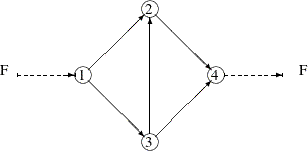The five roads connect four intersections illustrated by numbered nodes. Each minute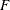vehicles enter and leave the network. Arc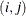refers to the road from intersectionto intersection, and the parameter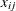refers to the flow fromto. The law that traffic flowing into each intersectionmust also flow out is described by the linear equality constraint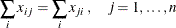In general, roads also have an upper capacity, which is the number of vehicles which can be handled per minute. The upper limits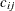can be enforced by boundary constraints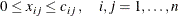Finding the maximum flow through a network is equivalent to solving a simple linear optimization problem, and for large problems, PROC LP or PROC NETFLOW can be used. The objective function is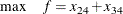and the constraints are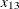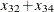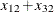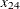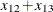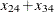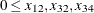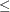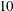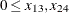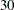The three linear equality constraints are linearly dependent. One of them is deleted automatically by the PROC NLP subroutines. Even though the default technique is used for this small example, any optimization subroutine can be used.

proc nlp all initial=.5;
max y;
parms x12 x13 x32 x24 x34;
bounds x12 <= 10,
x13 <= 30,
x32 <= 10,
x24 <= 30,
x34 <= 10;
/* what flows into an intersection must flow out */
lincon x13 = x32 + x34,
x12 + x32 = x24,
x24 + x34 = x12 + x13;
y = x24 + x34 + 0*x12 + 0*x13 + 0*x32;
run;


The iteration history is given in Output 7.9.2, and the optimal solution is given in Output 7.9.3.

Output 7.9.2: Iteration History

PROC NLP: Nonlinear Maximization

Newton-Raphson Ridge Optimization

Without Parameter Scaling

Iteration   Restarts Function
Calls
Active
Constraints
Objective
Function
Objective
Function
Change
Max Abs
Element
Ridge Ratio
Between
Actual
and
Predicted
Change
1 * 0 2 4   20.25000 19.2500 0.5774 0.0313 0.860
2 * 0 3 5   30.00000 9.7500 0 0.0313 1.683

Optimization Results
Iterations 2 Function Calls 4
Hessian Calls 3 Active Constraints 5
Objective Function 30 Max Abs Gradient Element 0
Ridge 0 Actual Over Pred Change 1.6834532374

 All parameters are actively constrained. Optimization cannot proceed.

Output 7.9.3: Optimization Results

PROC NLP: Nonlinear Maximization

Optimization Results
Parameter Estimates
Objective
Function
Active
Bound
Constraint
1 x12 10.000000 0 Upper BC
2 x13 20.000000 0
3 x32 10.000000 0 Upper BC
4 x24 20.000000 1.000000
5 x34 10.000000 1.000000 Upper BC

Value of Objective Function = 30

Finding a traffic pattern that minimizes the total delay to movevehicles per minute from node 1 to node 4 introduces nonlinearities that, in turn, demand nonlinear optimization techniques. As traffic volume increases, speed decreases. Let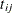be the travel time on arcand assume that the following formulas describe the travel time as decreasing functions of the amount of traffic: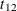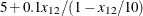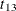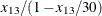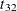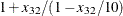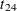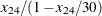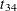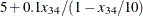These formulas use the road capacities (upper bounds), assuming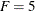vehicles per minute have to be moved through the network. The objective function is now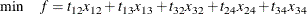and the constraints are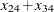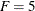Again, the default algorithm is used:

proc nlp all initial=.5;
min y;
parms x12 x13 x32 x24 x34;
bounds x12 x13 x32 x24 x34 >= 0;
lincon x13 = x32 + x34,  /* flow in = flow out */
x12 + x32 = x24,
x24 + x34 = 5;    /* = f = desired flow */
t12 = 5 + .1 * x12 / (1 - x12 / 10);
t13 = x13 / (1 - x13 / 30);
t32 = 1 + x32 / (1 - x32 / 10);
t24 = x24 / (1 - x24 / 30);
t34 = 5 + .1 * x34 / (1 - x34 / 10);
y = t12*x12 + t13*x13 + t32*x32 + t24*x24 + t34*x34;
run;


The iteration history is given in Output 7.9.4, and the optimal solution is given in Output 7.9.5.

Output 7.9.4: Iteration History

PROC NLP: Nonlinear Minimization

Newton-Raphson Ridge Optimization

Without Parameter Scaling

Iteration   Restarts Function
Calls
Active
Constraints
Objective
Function
Objective
Function
Change
Max Abs
Element
Ridge Ratio
Between
Actual
and
Predicted
Change
1   0 2 4   40.30303 0.3433 4.44E-16 0 0.508

Optimization Results
Iterations 1 Function Calls 3
Hessian Calls 2 Active Constraints 4
Objective Function 40.303030303 Max Abs Gradient Element 4.440892E-16
Ridge 0 Actual Over Pred Change 0.5083585587

 ABSGCONV convergence criterion satisfied.

Output 7.9.5: Optimization Results

PROC NLP: Nonlinear Minimization

Optimization Results
Parameter Estimates
Objective
Function
Active
Bound
Constraint
1 x12 2.500000 5.777778
2 x13 2.500000 5.702479
3 x32 -2.77556E-17 1.000000 Lower BC
4 x24 2.500000 5.702479
5 x34 2.500000 5.777778

Value of Objective Function = 40.303030303

The active constraints and corresponding Lagrange multiplier estimates (costs) are given in Output 7.9.6 and Output 7.9.7, respectively.

Output 7.9.6: Linear Constraints at Solution

PROC NLP: Nonlinear Minimization

Linear Constraints Evaluated at Solution
1 ACT 0 = 0 + 1.0000 * x13 - 1.0000 * x32 - 1.0000 * x34
2 ACT 4.4409E-16 = 0 + 1.0000 * x12 + 1.0000 * x32 - 1.0000 * x24
3 ACT 0 = -5.0000 + 1.0000 * x24 + 1.0000 * x34

Output 7.9.7: Lagrange Multipliers at Solution

PROC NLP: Nonlinear Minimization

First Order Lagrange Multipliers
Active Constraint Lagrange
Multiplier
Lower BC x32 0.924702
Linear EC  5.702479
Linear EC  5.777778
Linear EC  11.480257

Output 7.9.8 shows that the projected gradient is very small, satisfying the first-order optimality criterion.

Output 7.9.8: Projected Gradient at Solution

PROC NLP: Nonlinear Minimization

Free
Dimension
Projected